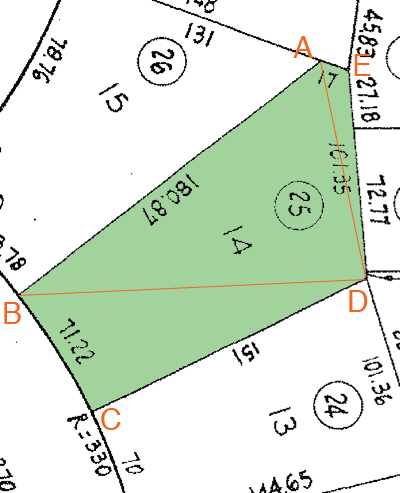SEARCH HOMEMath Central Quandaries & QueriesHello: I have been looking at your site, but I am still confused as to how to figure out the exact square footage of my irregular lot (since it is a gradual fan lot from the front, and triangles out in the back). I am including the measurements as well as the Property Plot Map (parcel number 25). These appear to be the Measurements: Front: 71.22 Left Side: 180.87 Back left Corner: 17 Back: 101.35 Right Side: 151 Thank you so much for your help. Sincerely, KarynHi Karyn,

I can find an approximation of the square footage of your lot which is based on the assumption that the diagram you sent us is approximately to scale. I added some labels to the diagram so that I can refer to it.I need to find the lengths of BD and DA and this is where I need to assume that the drawing is approximately to scale.

I printed the diagram after turning off any scaling on my printer. I then measured the lengths of AB, CD and DE in Centimeters as accurately as I could. Her is what I found.

Length (ft) Measurement (cm) Scale factor (ft/cm)
AB 180.87 10.1 180.87/10.1 = 17.91
CD 151 8.1 151/8.1 = 18.64
DE 101.35 5.6 101.35/5.6 = 18.10

These three scale factors should be the same. They are not but they are close so I found their average, 18.22 ft/cm and used it as a scale factor in order to approximate the lengths I need.

I next measured the lengths of BD and DA in centimeters on the diagram and multiplied each by 18.22 to approximate the lengths on your lot. I found the length of DB to be 167.59 feet and the length of DA to be 107.47 feet.

I then saw the lot as the quadrilateral ABCD and the triangle ADE. The area of the quadrilateral I found using the tool that Steve wrote. The calculated area is 14214 square feet. I then calculated the area of the triangle ADE using Heron's formula and found it to be 825 square feet. Thus my approximation of the area of your lot is 14214 + 825 = 15039 square feet.

HarleyMath Central is supported by the University of Regina and The Pacific Institute for the Mathematical Sciences.